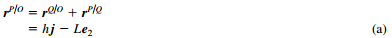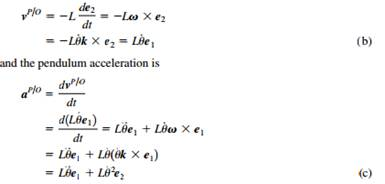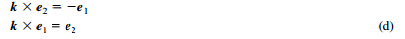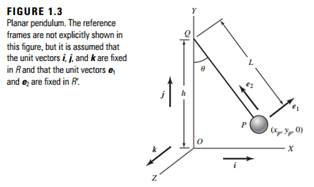### Create an Account

Home / Questions / Determine the kinetic energy of the planar pendulum of Example 11 EXAMPLE 11 Kinematics ...

# Determine the kinetic energy of the planar pendulum of Example 11 EXAMPLE 11 Kinematics of a planar pendulum Consider the planar pendulum shown in Figure 13 where the orthogonal unit vectors

Determine the kinetic energy of the planar pendulum of Example 1.1.

EXAMPLE 1.1

Kinematics of a planar pendulum

Consider the planar pendulum shown in Figure 1.3, where the orthogonal unit vectors e1 and e2 are fixed to the pendulum and they share the motion of the pendulum. The unit vectors i, j, and k, which point along the X, Y and Z directions, respectively, are fixed in time. The velocity and acceleration of the planar pendulum P with respect to point O are the quantities of interest. The position vector from point O to point P is written asMaking use of Eqs. (1.2) and (1.8) and noting that both h and L are constant with respect to time and the angular velocity v , the pendulum velocity isIn arriving at Eqs. (b) and (c), the following relations have been used.Jun 25 2020 View more View LessSubscribe To Get Solution